Publicité

# MAT 2B SR AREAS M01 INTRO(26 May 2016).ppt

26 Mar 2023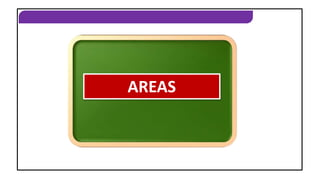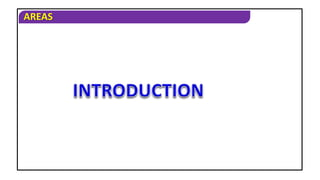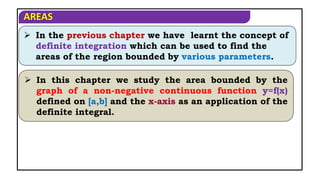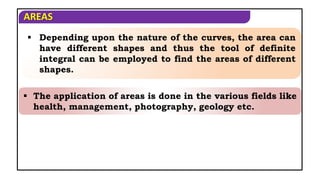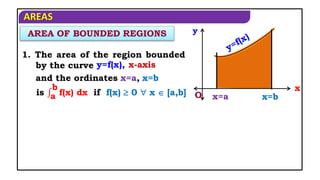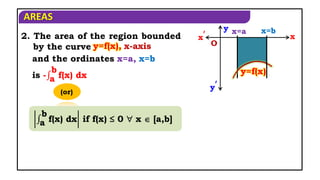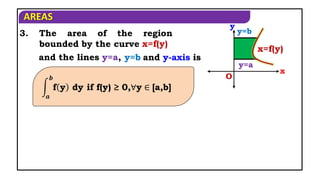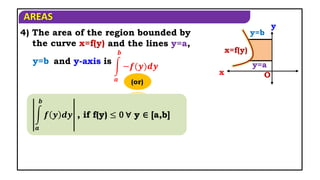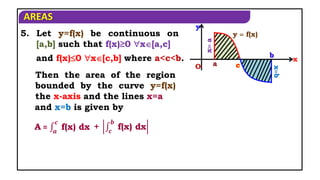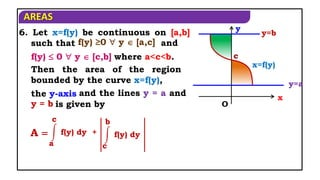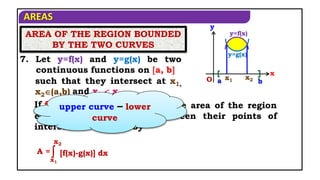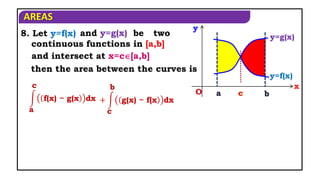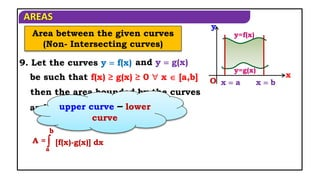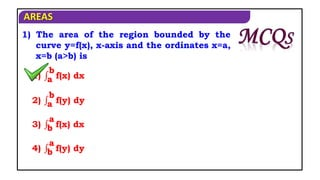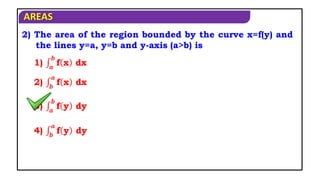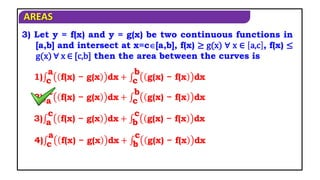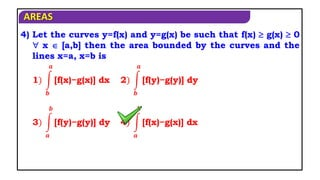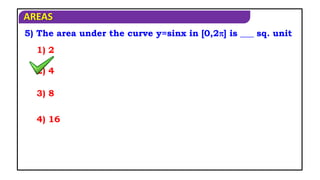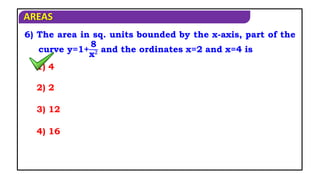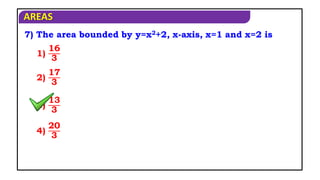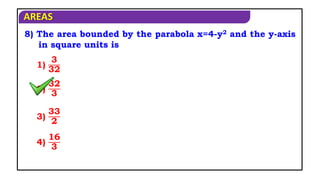1 sur 22
Publicité

### MAT 2B SR AREAS M01 INTRO(26 May 2016).ppt

1. AREAS AREAS
2. AREAS
3. AREAS  In the previous chapter we have learnt the concept of definite integration which can be used to find the areas of the region bounded by various parameters.  In this chapter we study the area bounded by the graph of a non-negative continuous function y=f(x) defined on [a,b] and the x-axis as an application of the definite integral.
4. AREAS  Depending upon the nature of the curves, the area can have different shapes and thus the tool of definite integral can be employed to find the areas of different shapes.  The application of areas is done in the various fields like health, management, photography, geology etc.
5. AREAS 1. The area of the region bounded by the curve AREA OF BOUNDED REGIONS x=b O x x=a y y=f(x), x-axis and the ordinates x=a, x=b is a b f(x) dx if f(x)  0  x  [a,b]
6. AREAS x x′ O y y′ y=f(x) 2. The area of the region bounded by the curve x=a x=b y=f(x), x-axis and the ordinates x=a, x=b is - a b f(x) dx (or) a b f(x) dx if f(x) ≤ 0  x  [a,b]
7. AREAS 3. The area of the region bounded by the curve y=a y=b x O y x=f(y) and the lines y=a, y=b and y-axis is 𝒂 𝒃 f y dy if f(y) ≥ 0,∀y ∈ [a,b] x=f(y)
8. AREAS 4) The area of the region bounded by the curve x=f(y) y=a y=b x O y x=f(y) and the lines y=a, y=b and y-axis is 𝒂 𝒃 −𝒇(𝒚)𝒅𝒚 (or) 𝒂 𝒃 𝒇 𝒚 𝒅𝒚 , if f(y) ≤ 0 ∀ y ∈ [a,b]
9. AREAS 5. Let y=f(x) be continuous on [a,b] such that f(x)≥0 x[a,c] Then the area of the region bounded by the curve y=f(x) the x-axis and the lines x=a x a xb y  f(x) y O x a b c and f(x)0 x[c,b] where a<c<b. and x=b is given by A = 𝒂 𝒄 f(x) dx + 𝒄 𝒃 f(x) dx
10. AREAS and the lines y = a and y = b 6. Let x=f(y) be continuous on [a,b] such that y=a y=b y O x c x=f(y) A  a c f(y) dy + c b f(y) dy f(y) ≥0  y  [a,c] and f(y)  0  y  [c,b] where a<c<b. Then the area of the region bounded by the curve x=f(y), the y-axis is given by
11. AREAS then the area of the region enclosed by the curves between their points of intersection is given by AREA OF THE REGION BOUNDED BY THE TWO CURVES y=f(x) y O x y=g(x) x1 x2 a b 7. Let y=f(x) and y=g(x) be two continuous functions on a, b such that they intersect at x1, x2(a,b) and x1  x2. If f(x)  g(x)  x  (x1,x2) upper curve – lower curve [f(x)-g(x)] dx A =  x1 x2
12. AREAS be two continuous functions in [a,b] 8. Let y=f(x) a c f(x) − g(x dx y=f(x) y O x y=g(x) a b c + c b g(x) − f(x dx and intersect at x=c[a,b] then the area between the curves is and y=g(x)
13. AREAS 9. Let the curves y  f(x) Area between the given curves (Non- Intersecting curves) y=g(x) O x y=f(x) x  b y x  a and y  g(x) be such that f(x) ≥ g(x) ≥ 0  x  [a,b] then the area bounded by the curves and the lines x=a, x=b is upper curve – lower curve [f(x)-g(x)] dx A =  a b
14. AREAS 1) The area of the region bounded by the curve y=f(x), x-axis and the ordinates x=a, x=b (a>b) is 1) a b f(x) dx 2) a b f(y) dy 3) b a f(x) dx 4) b a f(y) dy
15. AREAS 2) The area of the region bounded by the curve x=f(y) and the lines y=a, y=b and y-axis (a>b) is 1) 𝒂 𝒃 f x dx 2) 𝒃 𝒂 f x dx 3) 𝒂 𝒃 f y dy 4) 𝒃 𝒂 f y dy
16. AREAS 3) Let y = f(x) and y = g(x) be two continuous functions in [a,b] and intersect at x=c[a,b], f(x) ≥ g(x) ∀ x ∈ a,c , f(x) ≤ g(x) ∀ x ∈ [c,b] then the area between the curves is 1) c a f(x) − g(x dx + c b g(x) − f(x dx 2) a c f(x) − g(x dx + c b g(x) − f(x dx 3) a c f(x) − g(x dx + b c g(x) − f(x dx 4) c a f(x) − g(x dx + b c g(x) − f(x dx
17. AREAS 4) Let the curves y=f(x) and y=g(x) be such that f(x)  g(x)  0  x  [a,b] then the area bounded by the curves and the lines x=a, x=b is 1) 𝒃 𝒂 [f(x)−g(x)] dx 2) 𝒃 𝒂 [f(y)−g(y)] dy 3) 𝒂 𝒃 [f(y)−g(y)] dy 4) 𝒂 𝒃 [f(x)−g(x)] dx
18. AREAS 5) The area under the curve y=sinx in [0,2] is ___ sq. unit 1) 2 2) 4 3) 8 4) 16
19. AREAS 6) The area in sq. units bounded by the x-axis, part of the curve y=1+ 8 x𝟐 and the ordinates x=2 and x=4 is 1) 4 2) 2 3) 12 4) 16
20. AREAS 7) The area bounded by y=x2+2, x-axis, x=1 and x=2 is 1) 16 3 2) 17 3 3) 13 3 4) 20 3
21. AREAS 8) The area bounded by the parabola x=4-y2 and the y-axis in square units is 1) 3 32 2) 32 3 3) 33 2 4) 16 3
22. AREAS Thank you…
Publicité